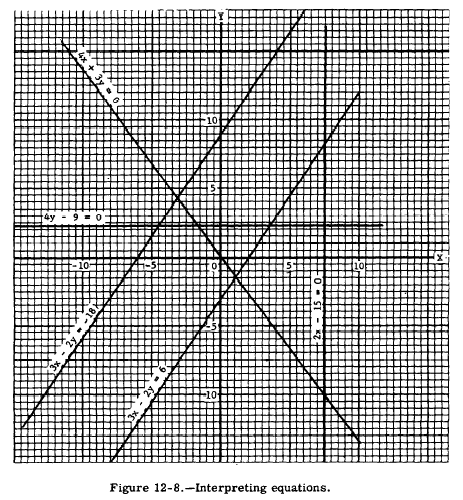Custom SearchINTERPRETING EQUATIONS  Recall that the general form for an equation in the first degree in one variable is ax + b = 0. The general form for first-degree equations in two variables is     ax + by + c =0 It is interesting and often useful to note what happens graphically when equations differ, in certain ways, from the general form. With this information, we know in advance certain facts concerning the equation in question. LINES PARALLEL TO THE AXES If in a linear equation the y term is missing, as in     2x - 15 = 0 the equation represents a line parallel to the Y axis and 7 1/2 units from it. Similarly, an equation such as     4y - 9 = 0 which has no x term, represents a line parallel to the X axis and 2 1/4 units from it. (See fig. 12-a.) The fact that one of the two variables does not appear in an equation means that there are no limitations on the values the missing variable can assume. When a variable does not appear, it can assume any value from zero to plus or minus infinity. This can happen only if the line represented by the equation lies parallel to the axis of the missing variable. Lines Passing Through the Origin A linear equation, such as     4x+3y=0 that has no constant term, represents a line passing through the origin. This fact is obvious since x = 0, y = 0 satisfies any equation not having a constant term. (See fig. 12-a.) Lines Parallel to Each Other An equation such as 3x-2y=6 has all possible terms present. It represents a line that is not parallel to an axis and does not pass through the origin. Equations that are exactly alike, except for the constant terms, represent parallel lines. As shown in figure 12-8, the lines represented by the equations 3x - 2y = -18 and 3x - 2y = 6 are parallel.Parallel lines have the same slope. Changing the constant term moves a line away from or toward the origin while its various positions remain parallel to one another. Notice in figure 12-8 that the line 3x - 2y = 6 lies closer to the origin than 3x - 2y = -18. This is revealed at sight for any pair of lines by comparing their constant terms. That one which has the constant term of greater absolute value will lie farther from the origin. In this case 3x - 2y = -18 will be farther from the origin since |-18| > |16|.. The fact that lines are parallel is indicated by the result when we try to solve two equations such as 3x - 2y = -18 and 3x - 2y = 6 simultaneously. Subtraction eliminates both x and y immediately. If both variables disappear, we cannot find values for them such that both equations are satisfied at the same time. This means that there is no solution. No solution implies that there is no point of intersection for the straight lines represented by the equations. Lines that do not intersect in the finite plane are parallel.Integrated Publishing, Inc. - A (SDVOSB) Service Disabled Veteran Owned Small Business